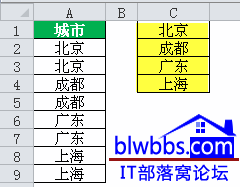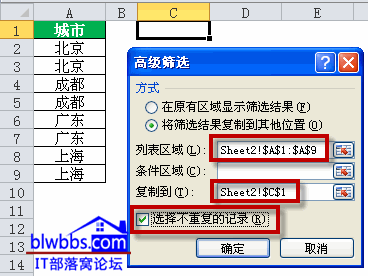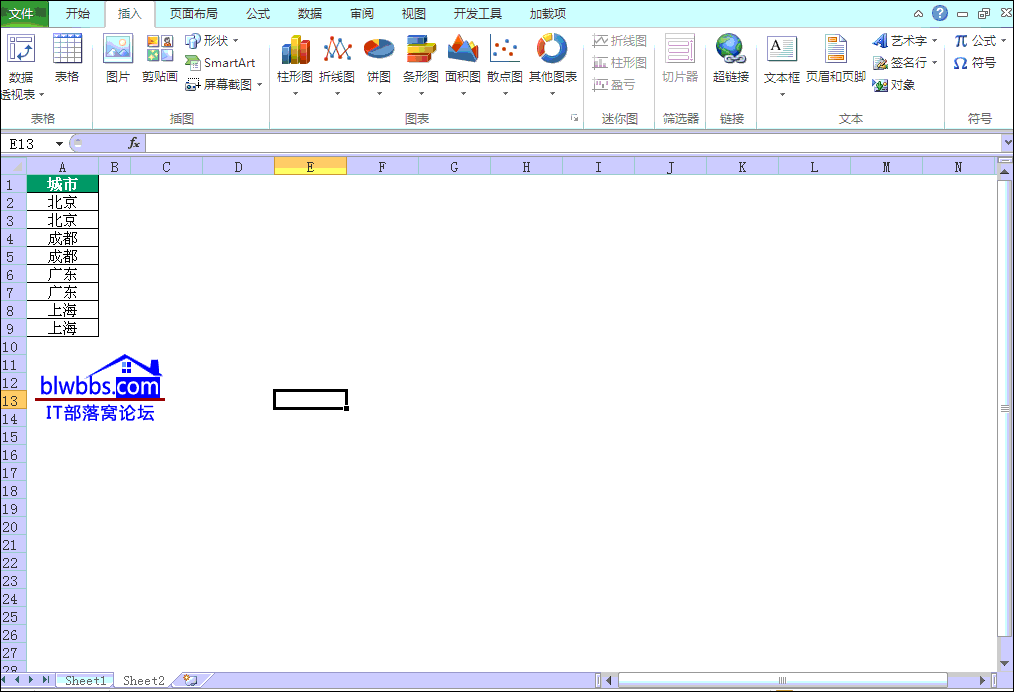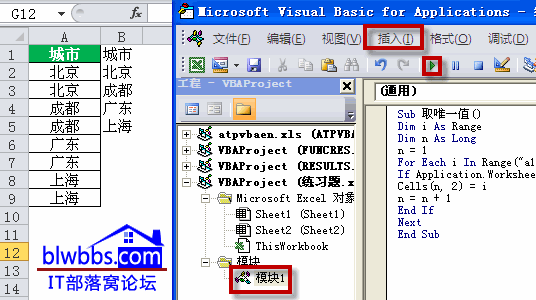# excel取唯一值的五种方法

[日期：2012-02-20]   来源：IT部落窝  作者：IT部落窝   阅读：18463[字体： ]

excel取唯一值，也称为提取不重复。在实际工作中，经常会使用到。

excel取唯一值的方法很多，比如通过高级筛选、函数公式以及自定义函数都可以实现。

我们以下图为例，A列城市，有重复的。需要提取唯一值放在C列。excel取唯一值方法一：

使用excel流行的筛选公式实现，C1输入公式：=INDEX(A:A,SMALL(IF(MATCH(A\$2:A\$9,A\$2:A\$9,)=ROW(\$1:\$8),ROW(\$2:\$9),4^8),ROW(A1)))&""，按ctrl+shift+enter组合键结束。

公式的思路和理解，请参看：Excel用函数公式筛选数据的方法

excel取唯一值方法二：

单击数据――高级筛选，可以直接筛选不重复值，即唯一值。使用高级筛选需要注意一点的是，必须包含列字段。比如下图A2：A9只是数据内容，需要再选中A1，即列表区域为：A1：A9。其实设置参看下图就OK。excel取唯一值方法三：

excel中数据透视表本身就有去重复功能。单击插入――数据透视表，然后勾选“城市”字段，唯一值就提取出来了。最后，也可以将数据透视表提取出来的唯一值复制到其余单元格，然后把不需要的数据透视表删得即可。

具体可以参看下面的动画演示，很简单的操作过程。excel取唯一值方法四：

学习VBA的朋友，也可以使用自定义函数取唯一值。

方法是：按Alt+F11组合键，插入→模块→在VBE窗口中复制以下代码，回到工作表中，C1单元格输入公式：=wsingle(\$A\$1:\$A\$9,ROW(A1))，下拉，直到出现0为止，A列所有的唯一不重复值就别提取出来了。

Function Wsingle(rng1 As Range, x As Integer)
Dim m As Range
Dim n As Range
Dim i As Integer
For Each m In rng1
k = k + 1
If k > Application.WorksheetFunction.CountA(rng1) + 10 Then Exit Function
If Application.WorksheetFunction.CountIf(rng1.Offset(k - 1, 0), m) = 1 Then
j = j + 1
If j = x Then
Wsingle = m
End If
End If
Next m
End Function

excel取唯一值方法五：

这个方法也是使用VBA代码，按Alt+F11组合键，插入→模块→在VBE窗口中复制以下代码，并单击工具栏的绿色“运行”按钮，就可以实现将A列唯一值提取自动提取到B列。

Sub 取唯一值()
Dim i As Range
Dim n As Long
n = 1
For Each i In Range("a1:a100")
If Application.WorksheetFunction.CountIf(Range("\$A\$1:" & i.Address), i) = 1 Then
Cells(n, 2) = i
n = n + 1
End If
Next
End Sub分享到： QQ空间 新浪微博 腾讯微博 人人网